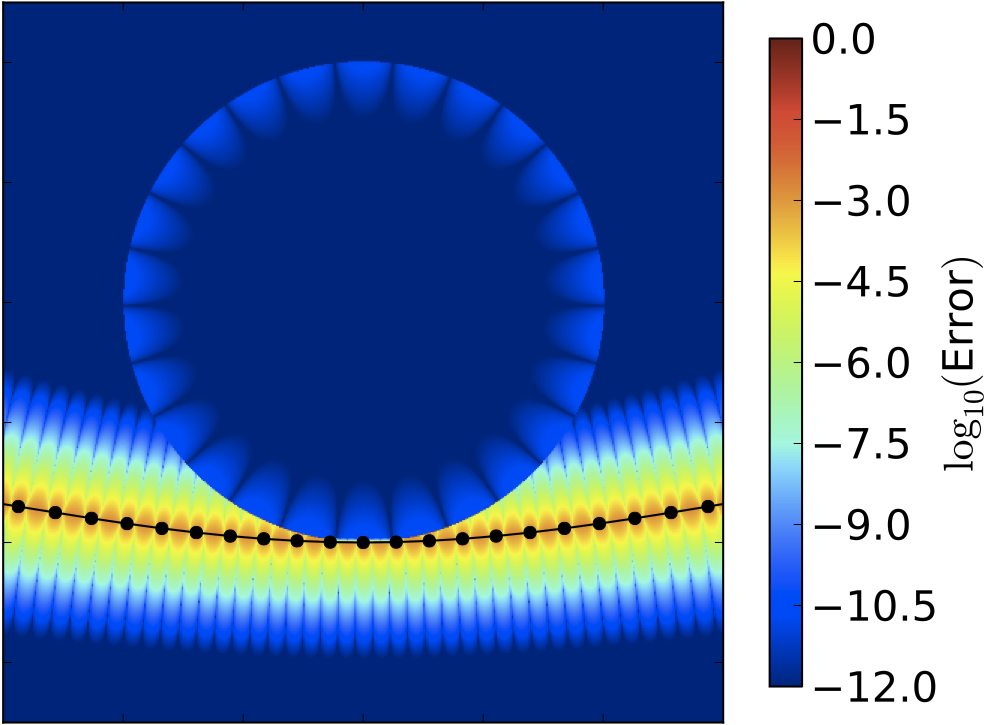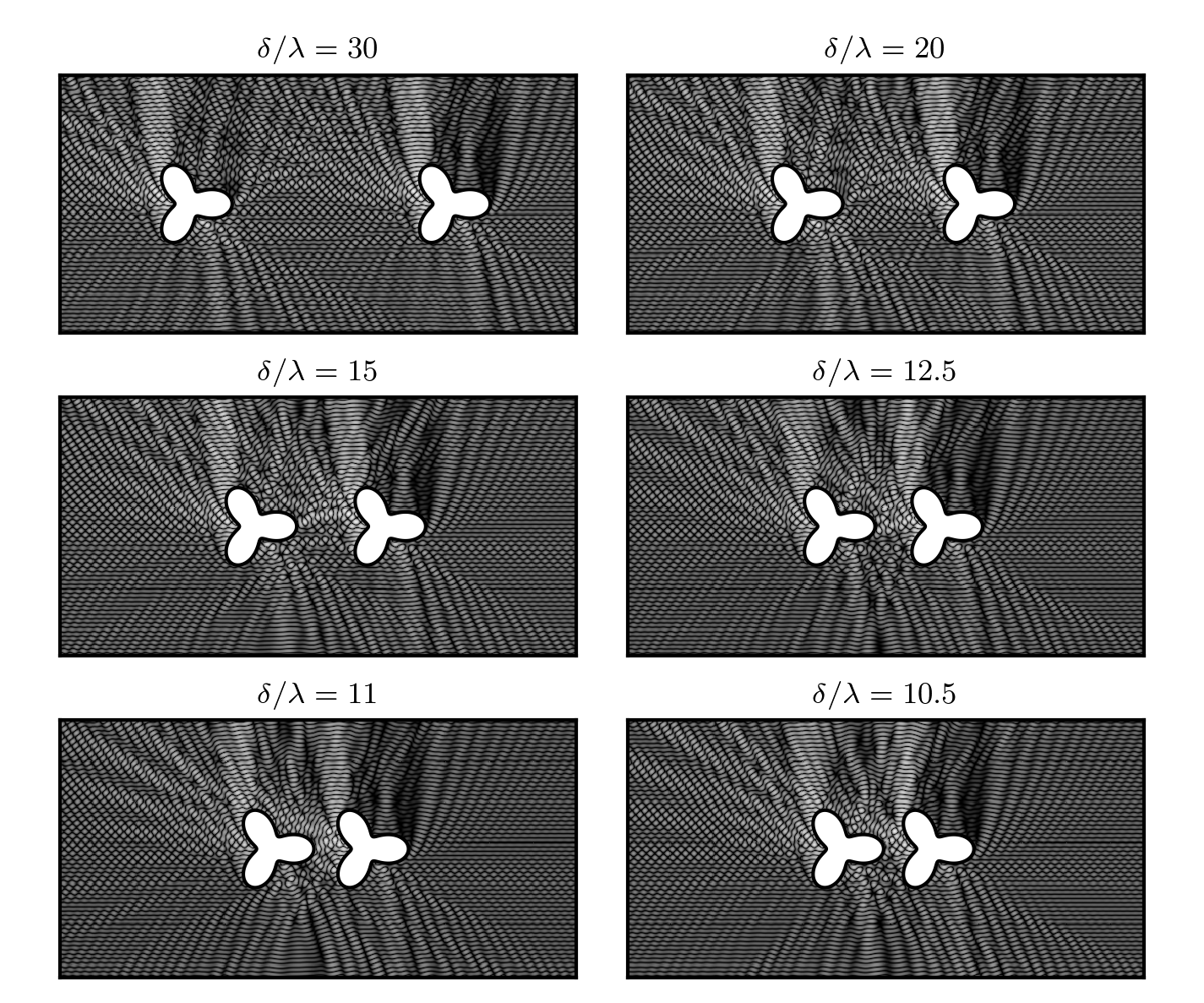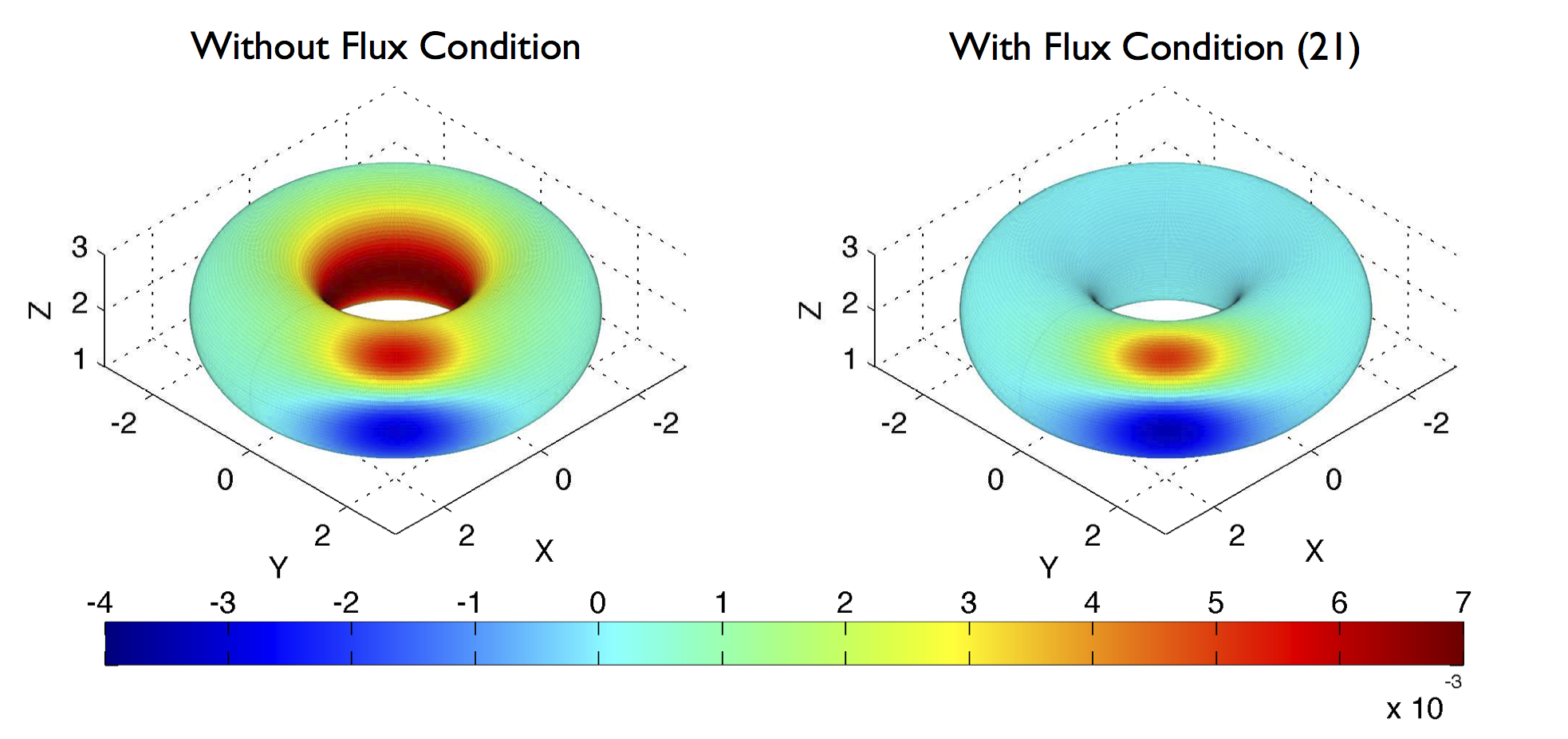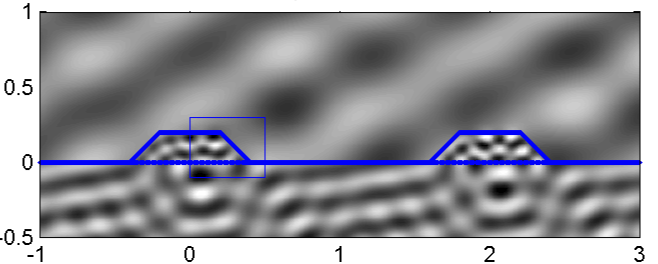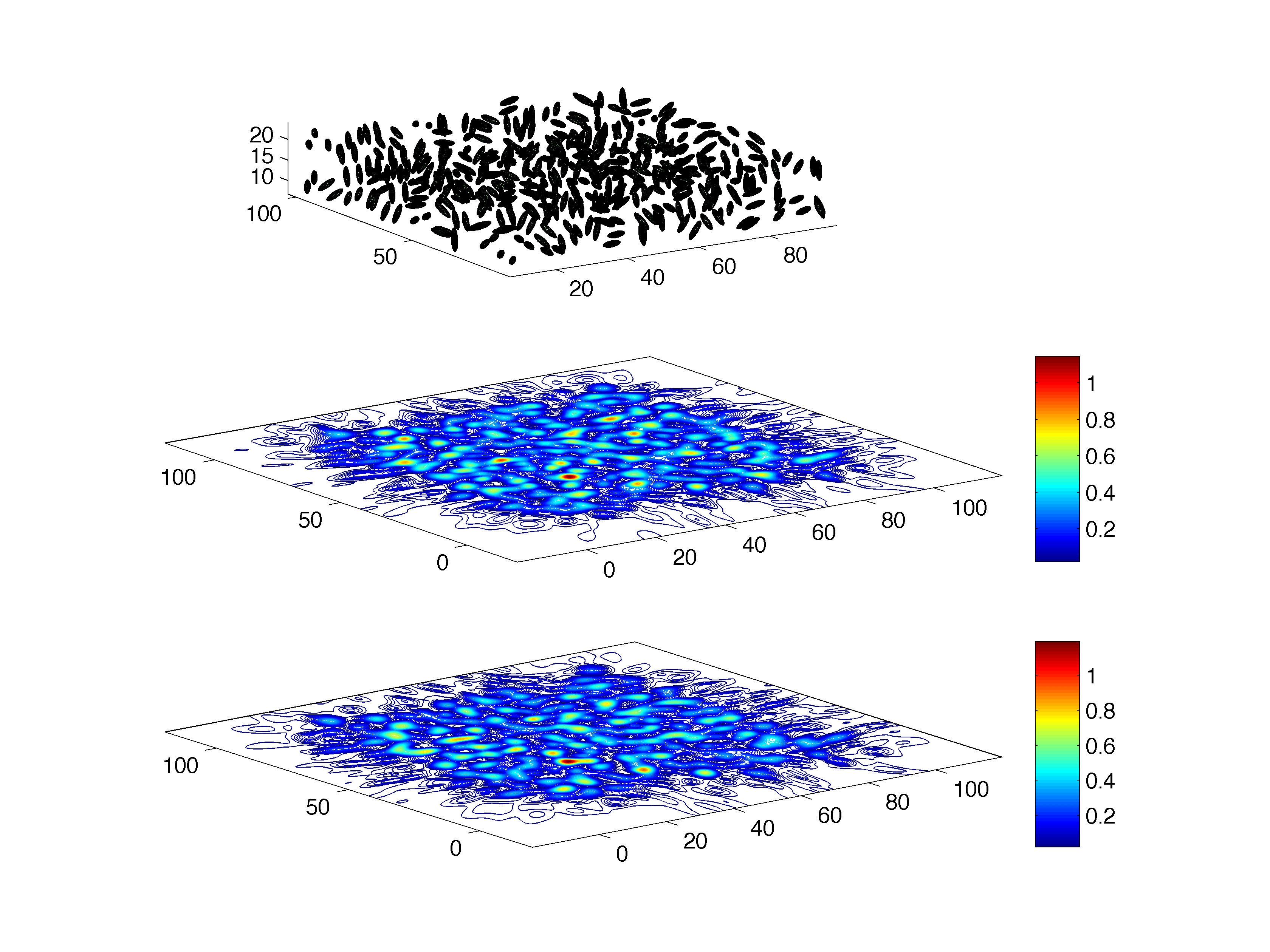# Research Projects in Electromagnetics

Background: One of the classical problems of mathematical physics concerns the solution of the time harmonic Maxwell equations in unbounded domains exterior to a complex geometry consisting of a mixture of metal and dielectric structures. This is an essential task in antenna design, radar scattering, analog and high-speed radio-frequency chip analysis, novel microstructured materials manufacturing, optics, and the study of electromagnetic compatibility. Despite many decades of work and much progress, current methods are still not fast enough or accurate enough for design by simulation, and not robust enough to be put in the hands of (non-expert) designers. Electrically large objects (those that are many wavelengths in size) are particularly difficult to deal with, especially when there are features present at sub-wavelength scales. As a result, there has been a concerted effort over many decades to develop numerical methods that can correctly predict the electromagnetic behavior of a given structure and, much more ambitiously, to allow engineers to alter the geometry during the design phase. Integral equation methods (sometimes called moment methods) have several crucial advantages. They impose the correct radiation condition at infinity without the need for an artificial boundary condition on a truncated computational domain and they are not subject to numerical dispersion errors.

State of the art: Historically, the numerical solution of the integral equations of scattering theory was dominated by the cost of solving the linear systems that result from their discretization. The fundamental obstacle is that the governing Green's function (in the frequency domain) is both oscillatory and slowly decaying, so that the linear systems which need to be solved are dense. Standard direct methods require O(n^3) operations where n is the number of points used in the discretization; this makes the modeling of "electrically large" and/or geometrically complex objects prohibitively expensive. Iterative solvers require O(n^2) operations, where the number of iterations depends on the condition number of the system being solved, a substantial improvement if the condition number is not too large. During the last decade or so, a new class of methods for the solution of the integral equations of scattering theory has appeared. The Fast Multipole Method (FMM), developed by the PI in collaboration with V. Rokhlin (Yale), with important contributions from many other research groups, is perhaps the most widely used of these methods:
• L. Greengard and V. Rokhlin, A fast algorithm for particle simulations, J. Comput. Phys., 73 , 325-348 (1987).
• W. C. Chew, J.-M. Jin, E. Michielssen, and J. Song, editors, Fast and Efficient Algorithms in Computational Electromagnetics, Artech House, Boston (2001).
• H. Cheng, W.Y. Crutchfield, Z. Gimbutas, L. Greengard, F. Ethridge, J. Huang, V. Rokhlin, N. Yarvin, and J. Zhao, A wideband fast multipole method for the Helmholtz equation in three dimensions, J. Comput. Phys., 216 , 300-325 (2006).
The FMM is a technique for reducing the cost of applying the integral operators of classical potential theory (electrostatics, gravitation, acoustics, electrodynamics, etc.) from O(n^2) to O(n) or O(n log n) . FMM-accelerated iterative methods reduce the cost of solving integral equations to O(n log n) and, to a large extent, have brought geometrically faithful numerical simulations in computational electromagnetics within practical reach. While implementations can always be improved, the FMM is now a fairly mature and robust technology. Nevertheless, a number of critical issues remain unresolved that prevent integral equations from realizing their full potential in enabling the goals of accurate prediction and design by simulation. These include the selection of an appropriate integral representation, the choice of the discretization scheme, and the development of suitable quadrature rules. Also critical will be fast direct solvers (in addition to FMM-based iterative ones), allowing the solution to a scattering problem to be rapidly updated when small changes are made to the geometry during a design process or the scattered field is sought from a fixed structure from multiple distinct directions of illumination.

Our group is working in all the above areas: Integral Equation Theory, including new methods for Multi-material interfaces , as well as Quadrature Methods, Fast Direct Solvers, and Fast Multipole Methods themselves.

 Quadrature methods Fast Direct Solvers